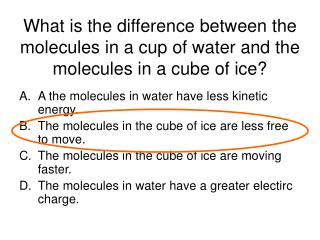DownloadDownload PresentationWhat is the difference between the molecules in a cup of water and the molecules in a cube of ice?

What is the difference between the molecules in a cup of water and the molecules in a cube of ice?

Télécharger la présentationWhat is the difference between the molecules in a cup of water and the molecules in a cube of ice?

- - - - - - - - - - - - - - - - - - - - - - - - - - - E N D - - - - - - - - - - - - - - - - - - - - - - - - - - -
Presentation Transcript

1. What is the difference between the molecules in a cup of water and the molecules in a cube of ice? • A the molecules in water have less kinetic energy. • The molecules in the cube of ice are less free to move. • The molecules in the cube of ice are moving faster. • The molecules in water have a greater electirc charge.

2. What phase change is occuring? • Is energy being absorbed or released? • Is this an endo or exothermic reaction A Melting Absorbed Endothermic

3. Phase Diagram A Pressure B C What is the phase of this substance at A? Temperature SOLID

4. Phase Diagram Pressure A B 1 atm C .2 atm If the pressure drops from 1 atm to .2 atm what phase change occurs at 0° C? 0° C Temperature Sublimation

5. Phase Diagram A Pressure B C What phase change occurs from B to C? Temperature Vaporization

6. What would be observed between points C and D? • Liquid and vapor • Solid and liquid • Only vapor • Only liquid 3 D C 2 1 A B

7. Which phase has the most kinetic energy • 1 • 2 • 3 • It is the same through out 3 D C 2 1 A B

8. What happens when a sealed rigid container of gas is heated? • The volume of the gas increases • The pressure of the gas decreases • The volume of the gas decreases • The pressure of the gas increases

9. According to Charle’s Law, what will happen to the piston when the gas is heated? • The piston will move up because the particles will get bigger • The piston will move down because there is more energy • The piston will move up because the particles are moving faster • There will be no change

10. C5H12 + 8 O2 5 CO2 + 6 H2O If 10 moles of O2 are reacted, how many moles of CO2 are produced. 10 mol O2 5 mol CO2 8 mol O2 6.25 moles CO2

11. If the air in an air mattress is at 50°C and a pressure of 1 atm, what is the pressure if the temperature rises to 70°C • P1 = 1 atm • T1 = 50°C • P2 = ? • T2 = 70°C P1 = P2 T1 T2 1.40 atm

12. A container has a volume of 15.6L, it contains a gas that exerts 47 kPa at 275 K, what is the volume of the container at STP? • P1 = 47 kPa • V1 = 15.6 L • T1 = 275 K • P2 = 101.3 kPa • V2 = ? • T2 = 273 K 7.19 L

13. 2 Al + 3 ZnCl2 2 AlCl3 + 3 Zn • How many grams of Zn are produced from 14 moles of ZnCl2 with excess Al? • 14 mol ZnCl2 3 mol Zn 65.39 g Zn 3 mol ZnCl2 1 mol Zn

14. 2 Al + 3 ZnCl2 2 AlCl3 + 3 Zn • How many Liters of Al are needed to produce 456g of AlCl3 456g AlCl3 1 mol AlCl3 2 mol Al 22.4 L Al 133.341 g AlCl3 2 mol AlCl3 1 mol Al 76.6 L Al